# PARENT FUNCTIONS PARENT FUNCTIONS Linear Quadratic Cubic Square

• Slides: 11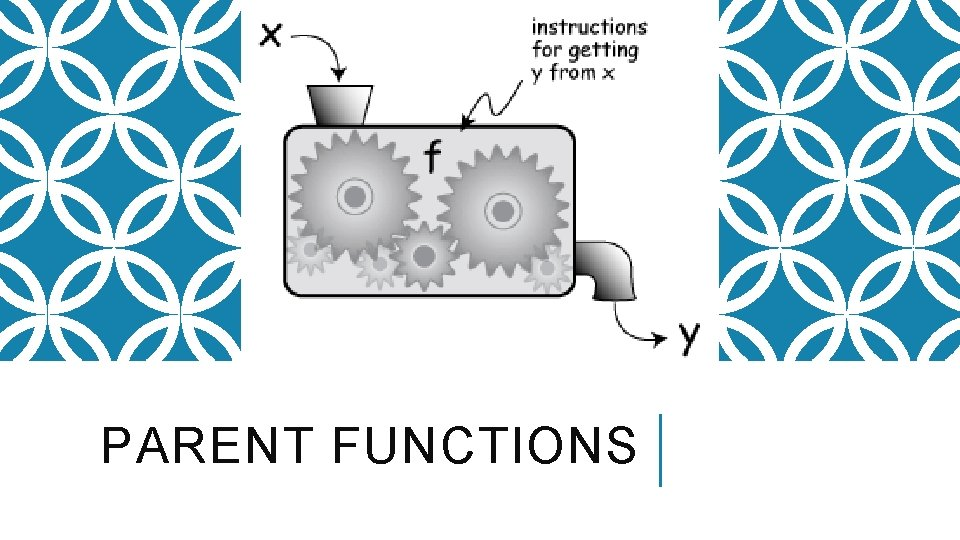PARENT FUNCTIONSPARENT FUNCTIONS Linear Quadratic Cubic Square Root Reciprocal Exponential Logarithmic Absolute ValueDOMAIN AND RANGE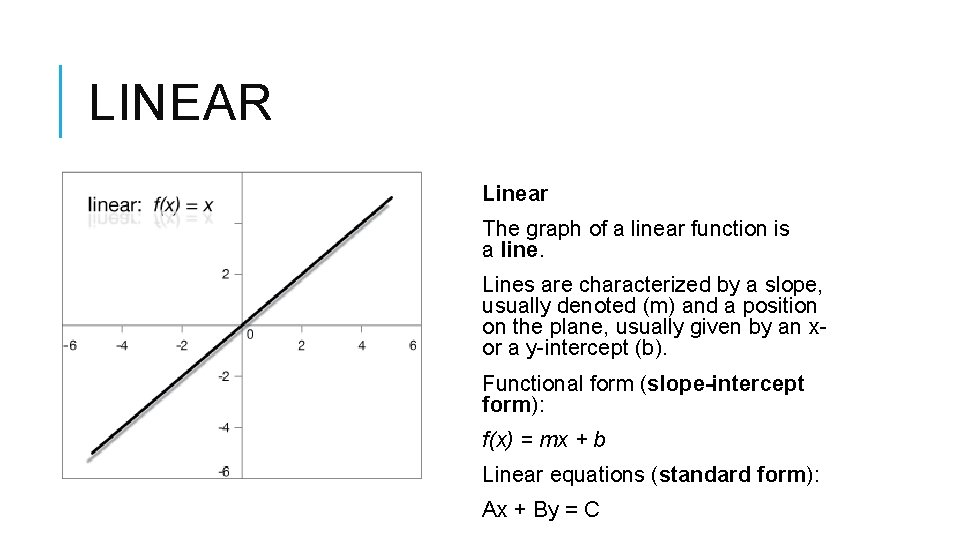LINEAR Linear The graph of a linear function is a line. Lines are characterized by a slope, usually denoted (m) and a position on the plane, usually given by an x- or a y-intercept (b). Functional form (slope-intercept form): f(x) = mx + b Linear equations (standard form): Ax + By = C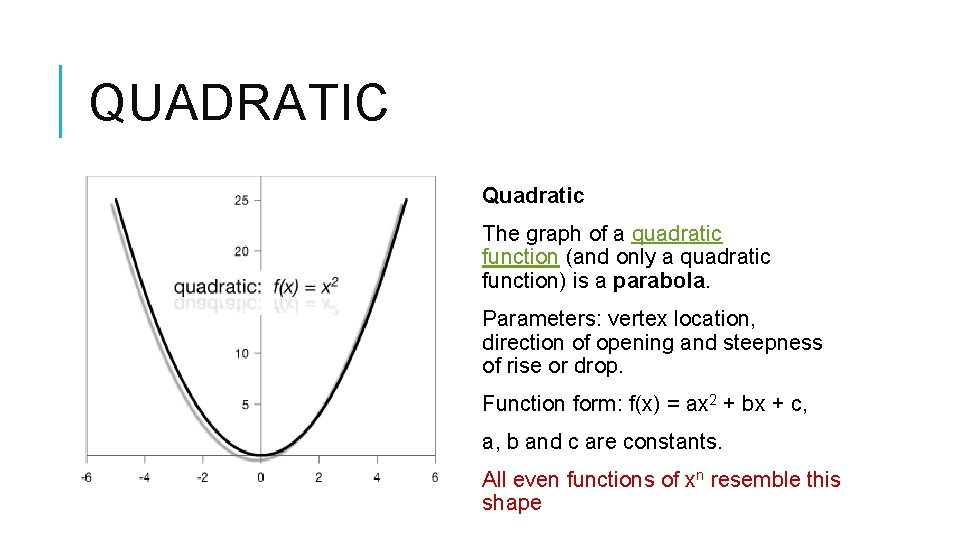QUADRATIC Quadratic The graph of a quadratic function (and only a quadratic function) is a parabola. Parameters: vertex location, direction of opening and steepness of rise or drop. Function form: f(x) = ax 2 + bx + c, a, b and c are constants. All even functions of xn resemble this shape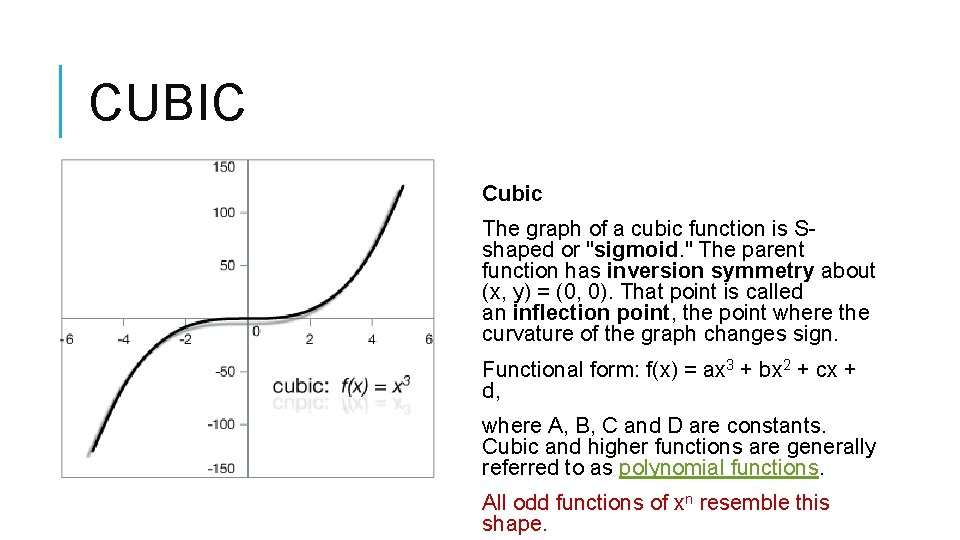CUBIC Cubic The graph of a cubic function is Sshaped or "sigmoid. " The parent function has inversion symmetry about (x, y) = (0, 0). That point is called an inflection point, the point where the curvature of the graph changes sign. Functional form: f(x) = ax 3 + bx 2 + cx + d, where A, B, C and D are constants. Cubic and higher functions are generally referred to as polynomial functions. All odd functions of xn resemble this shape.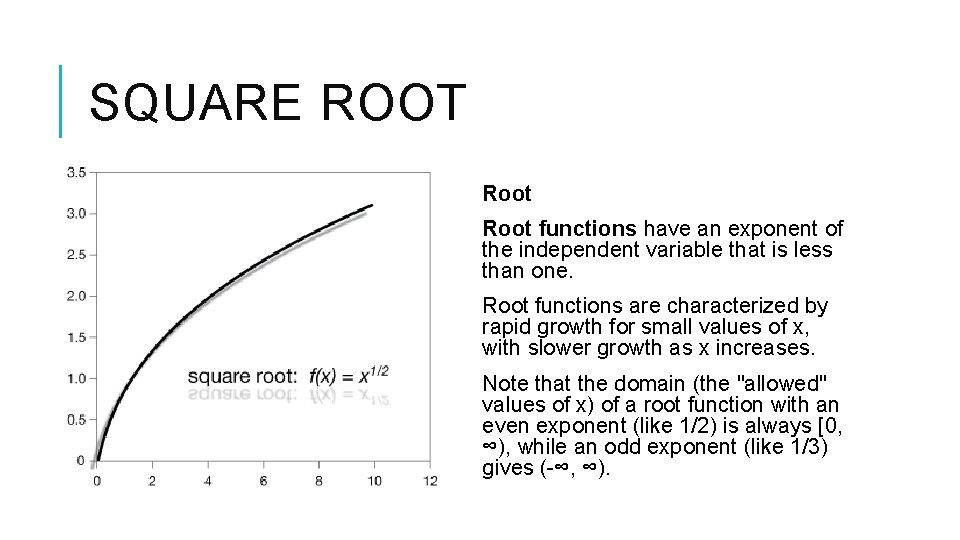SQUARE ROOT Root functions have an exponent of the independent variable that is less than one. Root functions are characterized by rapid growth for small values of x, with slower growth as x increases. Note that the domain (the "allowed" values of x) of a root function with an even exponent (like 1/2) is always [0, ∞), while an odd exponent (like 1/3) gives (-∞, ∞).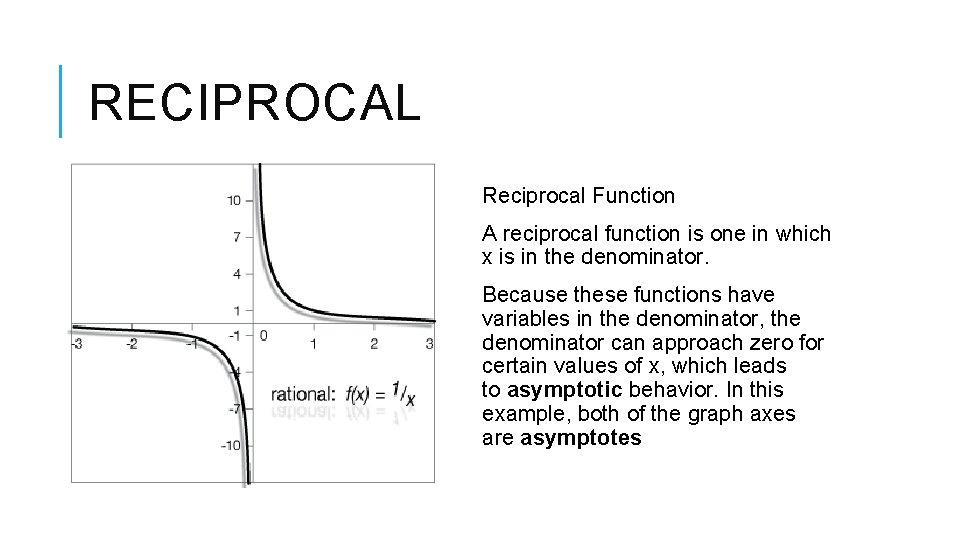RECIPROCAL Reciprocal Function A reciprocal function is one in which x is in the denominator. Because these functions have variables in the denominator, the denominator can approach zero for certain values of x, which leads to asymptotic behavior. In this example, both of the graph axes are asymptotesEXPONENTIAL Exponential In an exponential function, the independent variable is in the exponent, thus exponential functions grow very rapidly compared to polynomial functions.LOGARITHMIC Logarithmic functions are the inverses of exponential functions. They grow continuously as the independent variable grows, but the rate of growth diminishes, too.ABSOLUTE VALUE Absolute values carry the magnitude of the value, regardless of whether the value is positive or negative. This means that absolute values are always positive. They can usually be characterized in a graph by the sharp corner in the function.